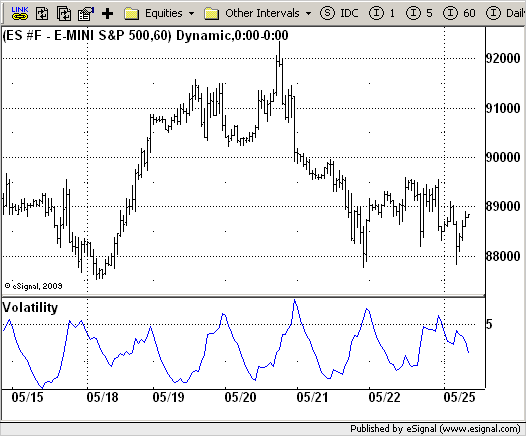# Volatility

ICE Data Services -

Volatility.efs
EFSLibrary - Discussion Board

File Name: Volatility.efs

Description:
Volatility

Formula Parameters:

• nLength : 10

Notes:
The Volatility function measures the market volatility by plotting a smoothed average of the True Range. It returns an average of the TrueRange over a specific number of bars, giving higher weight to the TrueRange of the most recent bar.

Volatility.efsEFS Code:

```/*********************************
Provided By:
eSignal (Copyright c eSignal), a division of Interactive Data
Formula Script (EFS) is for educational purposes only and may be
modified and saved under a new file name.  eSignal is not responsible
for the functionality once modified.  eSignal reserves the right
to modify and overwrite this EFS file with each new release.

Description:
Volatility

Version:            1.0  05/22/2009

Formula Parameters:                     Default:
nLength                             10

Notes:
The Volatility function measures the market volatility by plotting a
smoothed average of the True Range. It returns an average of the TrueRange
over a specific number of bars, giving higher weight to the TrueRange of
the most recent bar.

**********************************/
var fpArray = new Array();
var bInit = false;

function preMain() {
setStudyTitle("Volatility");
setCursorLabelName("Volatility");
var x = 0;
fpArray[x] = new FunctionParameter("nLength", FunctionParameter.NUMBER);
with(fpArray[x++]) {
setLowerLimit(1);
setDefault(10);
}
}

var xVolatility = null;

function main(nLength){
var nBarState = getBarState();
var nVolatility = 0;
if (nBarState == BARSTATE_ALLBARS) {
if (nLength == null) nLength = 10;
}
if (bInit == false) {
xVolatility = efsInternal("Calc_Volatility", nLength);
bInit = true;
}
nVolatility = xVolatility.getValue(0);
if (nVolatility == null) return;
return nVolatility;
}

var bSecondInit = false;
var xATR = null;

function Calc_Volatility(nLength) {
var nRes = 0;
var nATR = 0;
var nRef = ref(-1);
if (bSecondInit == false) {
xATR = atr(nLength);
bSecondInit = true;
}
nATR = xATR.getValue(0);
if (nATR == null) return;
if (nRef == null) {
nRes = nATR;
} else {
nRes = ((nLength - 1) * nRef + nATR) / nLength;
}
return nATR;
}```